## Saturday, September 14, 2013

### Directions

The following problem has a question and two statements which are labeled (1) and (2) in which certain data are given. You have to decide whether the data given in the statements are sufficient for answering the question. Using the data given in the problem plus your knowledge on mathematics and every day facts, choose:

(1)    If you can get the answer from (1) ALONE but not from (2) alone
(2)    If you can get the answer from (2) ALONE but not from (1) alone
(3)    If you can get the answer from BOTH (1) and (2) TOGETHER, but not from (1) alone or (2) alone
(4)    If EITHER statement (1) ALONE or statement (2) ALONE suffices
(5)    If you CANNOT get the answer from statements (1) and (2) TOGETHER, but need even more data

Question
A jar is filled with 60 marbles. All the marbles in the jar are either red or green. What is the smallest number of marbles that must be drawn from the jar in order to be certain that a red marble is drawn?
(1)    The  ratio of red marbles to green marbles is 2 : 1
(2)    There are 20 green marbles in the jar

Correct Answer – Choice 4. Each statement is independently sufficient.

Information given in the question stem
1.    Total of 60 marbles.
2.    Only red and green marbles.

Statement 1:  Ratio of red to green marbles is 2 : 1.
There are 40 red marbles and 20 green marbles. The smallest number of marbles that must be drawn from the jar to be certain that a red marble is drawn is when you can be certain that if the all the marbles that you have drawn to that point are green and that there are no more green marbles.

i.e., the 21st marble will certainly be a red marble. Statement 1 provides us the required data.
Statement 1 is SUFFICIENT.

Statement 2
: There are 20 green marbles in the jar.
Statement 2 directly gives the number of green marbles. As explained in statement 1 this information is sufficient to answer the least number of marbles required to be certain that a red marble is drawn.

Statement 2 is also SUFFICIENT.

Choice (4) is the correct answer.

### Directions

The following problem has a question and two statements which are labeled (1) and (2) in which certain data are given. You have to decide whether the data given in the statements are sufficient for answering the question. Using the data given in the problem plus your knowledge on mathematics and every day facts, choose:

(1)    If you can get the answer from (1) ALONE but not from (2) alone
(2)    If you can get the answer from (2) ALONE but not from (1) alone
(3)    If you can get the answer from BOTH (1) and (2) TOGETHER, but not from (1) alone or (2) alone
(4)    If EITHER statement (1) ALONE or statement (2) ALONE suffices
(5)    If you CANNOT get the answer from statements (1) and (2) TOGETHER, but need even more data

Question

Plane X files at r miles per hour from A to B. Plane Y files at S miles per hour from B to A. Both planes take off at the same time. Which plane files at a faster rate? Town C is between A and B.
(1)    C is closer to A than it is to B
(2)    Plane X flies over C before plane Y

Correct Answer – Choice 5. Data is INSUFFICIENT

Information given in the question stem
1.    Plane X and Plane Y take off at the same time.
2.    Town C is between A and B.

Statement 1: C is closer to A than it is to B

This statement is not helpful in determining which of the two planes is faster unless we know that the two planes met at C. That is not stated in the statement or the question stem.

Statement 1 is INSUFFICIENT.

Statement 2: Plane X flies over C before Plane Y

This statement alone is not sufficient to determine which of the planes is faster unless we know where C is located.

Statement 2 is INSUFFICIENT.

Combining the two statements, we know that C closer to A. X flies over C before Y.

Because C is closer to A, even if X is as fast as Y or even if it is slower than Y, X could have flown over C earlier than Y.

On the contrary if the statement had mentioned that Y flew over C before X and C is closer to A than to B, we could have inferred that Y was faster than X.

Even after using the two statements we cannot infer which plane is faster.

Choice 5 is the correct answer.

## Wednesday, February 20, 2013

### TANCET 2012 Quant - Qn 43

This question tests your ability to express one variable in terms of the other. Level of difficutly easy.

If x = 2k – 2 and y = 4k^2, then what is y in terms of x?
1. x + 2
2. ((x + 2)^2)/2
3. (x + 2)^2
4.  x^2 + 4
5. (x + 4)^2

## Friday, February 8, 2013

### TANCET 2012 Quant - Percents - Q42

This question appeared in the Quant section of the TANCET 2012 Paper. An easy percents question.

Question

If S is 150 percent of T, then T is what percent of S + T?
1. 33.33%
2. 40%
3. 75%
4. 80%
5. 85%

The easiest way to solve this question is by assuming a value of T.

Take T to be 100.

Therefore, S = 150% of T = 150% of 100 = 150.

So, S + T = 150 + 100 = 250.

We need to find out T as a percent of S + T

i.e., (100 / 250)* 100 = 40%

## Monday, November 26, 2012

### TANCET 2008 Quant Qn 9 - Geometry, Mensuration

Question 9 : Geometry - Right Triangles : If the shaded area is one half the area of triangle ABC and angle ABC is right angle, then the length of line segment AD is

1.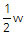2. c
3.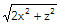4.5.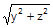## Thursday, February 2, 2012

### TANCET Previous Year Question Papers

I am putting together answers for previous year TANCET MBA question papers. I have started with the quant section of the TANCET 2008 paper.

Here is the set of links to all the questions with explanatory answers.

Quant Section of TANCET 2008
1. Question 1 : Linear Equation : An owner of a pizza stand sold small slices of pizza for Rs. 150 each and large slices for Rs. 250 each. One night he sold 5000 slices, for a total of Rs. 10.50 lakh. How many small slices were sold?
2. Question 2 : Framing Equations : Jack has three more cards than Bill. Together they have 47 cards. If x represents the number of cards Bill has, then an equation that can be used to determine the number of cards each one has is
3. Question 3 : Number Properties - Fractions : If two fractions, each of which has a value between 0 and 1, are multiplied together, the product will be:
4. Question 4 : Work Time : A father can do a certain job in x hours. His son takes twice as long to do the job. Working together, they can do the job in 6 hours. How many hours does the father take to do the job?
5. Question 5 : Mensuration : If x units are added to the length of the radius of a circle, what is the number of units by which the circumference of the circle is increased?
6. Question 6 : Percents, Comparison : John weighs twice as much as Maria. Marcia's weight is 60% of Bob's weight. Dave weighs 50% of Lee's weight. Lee weighs 190% of John's weight. Which of these 5 persons weighs the least?
7. Question 7 : Mathematical Expressions : There were P people in a room when a meeting started. Q people left the room during the first hour, while R people entered the room during the same time. What expression gives the number of people in the room after the first hour as a percentage of the number of people in the room who have been there since the meeting started?
8. Question 8 : Mathematical Expressions : It costs Rs. x each to make the first thousand copies of a compact disk and Rs. y to make each subsequent copy. If z is greater than 1,000, how many Rupees will it cost to make z copies of the compact disk?
9. Question 9 : Geometry - Right Triangles : If the shaded area is one half the area of triangle ABC and angle ABC is right angle, then the length of line segment AD is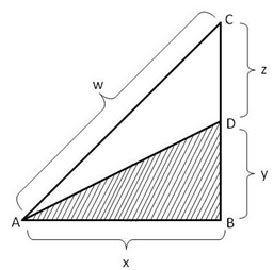10. Question 10 : Speed, Distance & Time : A plane flying north at 500 kmph passes over a city at 12 noon. A plane flying east at the same attitude passes over the same city at 12.30 pm. The plane is flying east at 400 kmph. To the nearest hundred km, how far apart are the two planes at 2 pm?
11. Question 11 : Framing Expressions : Profits : A clothing manufacturer has determined that she can sell 100 suits a week at a selling price of Rs. 200 each. For each rise of Rs. 4 in the selling price she will sell 2 less suits a week. If she sells the suits for Rs. x each, how many rupees a week will she receive from the sales of the suits?

12. Question 12 : Framing Expressions : Set Theory : The schedule of G first year students was inspected. It was found that M were taking a Mathematics course, L were taking a Language course and B were taking both a Mathematics course and a Language course. Which of the following expression gives the percentage of the students whose schedule was inspected who were taking neither a mathematics course nor a language course?

13. Question 13 : Speed, Distance & Time : A plane flying north at 500 kmph passes over a city at 12 noon. A plane flying east at the same attitude passes over the same city at 12.30 pm. The plane is flying east at 400 kmph. To the nearest hundred km, how far apart are the two planes at 2 pm?

14. Question 14 : Percents » Joan started work 2 years ago. Her starting salary was half of Mike's salary at that time. Each year since then Joan and Mike have received a rise of 10% in their respective salary. What percentage (to the nearest percent) of Mike's current salary is Joan's current salary?

15. Question 15 : Mensuration - Solid Geometry » ABCD has area equal to 28. BC is parallel to AD. BA is perpendicular to AD. If BC is 6 and AD is 8, then what is CD?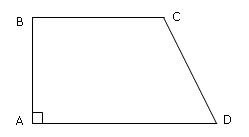16. Question 16 : Mensuration - Solid Geometry » Which of the following figures has the largest area?
1. A circle of radius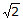2. An equilateral triangle whose sides each have length 4
3. A triangle whose sides have lengths 3, 4 and 5

17. Question 17 : Mathematical Expressions - equations » A charity solicited P persons over the phone who agreed to an average pledge of Rs.R each. Q of these people who had pledged an average of Rs. S each never sent in the pledged amount. Which of the following expressions represents the percentage of pledged money that the charity received?

18. Question 18 : Number Theory » If x, y, z are chosen from the three numbers, -3,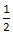and 2, what is the largest possible value of the expression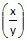z2?

19. Question 19 : Mensuration - Solid Geometry » The hexagon ABCDEF is regular. That means all its sides are of the same length and all its interior angles are of the same size. Each side of the hexagon is 2m. What is the area of the rectangle BCEF?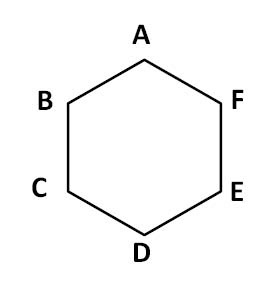Answer and Explanation »

20. Question 20 : Permutation » 36 identical chairs must be arranged in rows with the same number of chairs in each row. Each row must contain at least three chairs and there must be at least three rows. A row is parallel to the front of the room. How many different arrangements are possible?

## Thursday, January 26, 2012

### Profit Loss Question

Profit Loss is a relatively easy topic and quite often gets tested in these kinds of entrance tests.

Question